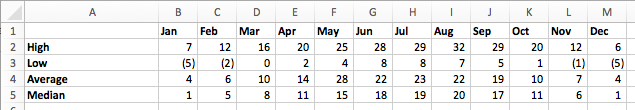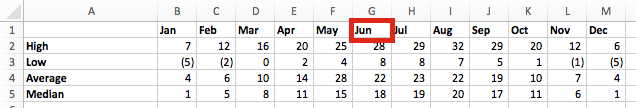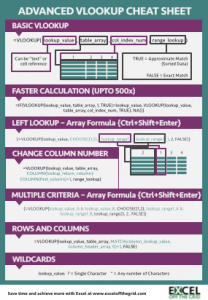Get our FREE VBA eBook of the 30 most useful Excel VBA macros.

Automate Excel so that you can save time and stop doing the jobs a trained monkey could do.

# How to use VLOOKUP with columns and rowsThere are times when our data is laid out in columns and rows.  In these circumstances, we may need to look up to both the column and the row at the same time, such as in the data below.But how can we achieve this?

## VLOOKUP & MATCH

By combining the VLOOKUP function with the MATCH function, we can achieve a lookup to a row and a column at the same time; this is often referred to as a two-way lookup.

### MATCH

The MATCH function is a very useful; it returns the position of a lookup value within a range.

Using our example data; we can find the column number of “Jun” using the Match function.`=MATCH("Jun",B1:M1,0)`

The result of this formula is 6, as in the Range B1-M1 “Jun” is the 6th item.  If we were to look up “Nov”, this would return 11, as that is the 11th item.

The last argument in the MATCH function is important.  We will be using 0 as that will provide an exact match.

### VLOOKUP & MATCH together

We can insert MATCH into the VLOOKUP function in place of the column number.

The VLOOKUP function counts the first column as 1, but our MATCH function starts at column B, so it is necessary to add 1 to the column number for the VLOOKUP to return the value from the correct column.The formula in B12 is as follows:

`=VLOOKUP(B9,A2:M5,MATCH(B10,B1:M1,0)+1,FALSE)`

## Looking up multiple rows

We’ve seen, in previous posts, that it is possible to use VLOOKUP with multiple criteria where the data is in two or more columns.  But what if we want to match multiple rows?

The example below shows July appearing twice in our data, once for 2016 and once for 2017.  By making the MATCH formula an array formula we can match the two column criteria of month and year together.The formula in cell B14 is:

`{=VLOOKUP(B10,A3:N6,MATCH(B11&"^"&B12,B1:N1&"^"&B2:N2,0)+1,FALSE)}`

This formula is starting to look a bit complicated now, so let’s break it down.

Firstly, this is an array formula.  Type the formula into Excel without the { }, but press Ctrl+Alt+Enter to enter the formula.  Excel will then add the { } by itself automatically.

`{=VLOOKUP(B10,A3:N6,MATCH(B11&"^"&B12,B1:N1&"^"&B2:N2,0)+1,FALSE)}`

Secondly, let’s just look at the first argument of the MATCH function.  This is simply combining the values of “Jul” and “2016” together with a spacer character in the middle.

`{=VLOOKUP(B10,A3:N6,MATCH(B11&"^"&B12,B1:N1&"^"&B2:N2,0)+1,FALSE)}`

The next argument of the MATCH function creates a temporary array of values with a spacer character in the middle.  This only works because it is an array formula.

`{=VLOOKUP(B10,A3:N6,MATCH(B11&"^"&B12,B1:N1&"^"&B2:N2,0)+1,FALSE)}`

The temporary array would include the following {“Jul^2016” , “Aug^2016” , “Sep^2016” , “Oct^2016” […all the way up to…] “Jun^2017” , “Jul^2017”}

The lookup value in the MATCH function is compared to this temporary array.  Provided the year and the month match a value will be returned.  By changing the year in cell B12 the value from N5, rather than B5 will be returned.  The image below shows the result as 27, rather than 23.## Multiple condition rows and columns

If you ever need to match multiple condition rows and multiple condition columns together, then it’s probably best to consider the INDEX/MATCH/MATCH formula.  As I’m not sure it’s possible to push the VLOOKUP formula that far.

Download the Advanced VLOOKUP Cheat Sheet.  It includes most of the tips and tricks we’ve covered in this series, including faster calculations, multiple criteria, left lookup and much more.

Please download it and pin it up at work, you can even forward it onto your friends and co-workers.## Other posts in the Mastering VLOOKUP SeriesGet our FREE VBA eBook of the 30 most useful Excel VBA macros.

Automate Excel so that you can save time and stop doing the jobs a trained monkey could do.

Don’t forget:

If you’ve found this post useful, or if you have a better approach, then please leave a comment below.

Do you need help adapting this to your needs?

I’m guessing the examples in this post didn’t exactly meet your situation.  We all use Excel differently, so it’s impossible to write a post that will meet everybody’s needs.  By taking the time to understand the techniques and principles in this post (and elsewhere on this site) you should be able to adapt it to your needs.

But, if you’re still struggling you should:

1. Read other blogs, or watch YouTube videos on the same topic.  You will benefit much more by discovering your own solutions.
2. Ask the ‘Excel Ninja’ in your office.  It’s amazing what things other people know.
3. Ask a question in a forum like Mr Excel, or the Microsoft Answers Community.  Remember, the people on these forums are generally giving their time for free.  So take care to craft your question, make sure it’s clear and concise.  List all the things you’ve tried, and provide screenshots, code segments and example workbooks.
4. Use Excel Rescue, who are my consultancy partner.   They help by providing solutions to smaller Excel problems.

What next?
Don’t go yet, there is plenty more to learn on Excel Off The Grid.  Check out the latest posts:

Save

## 3 thoughts on “How to use VLOOKUP with columns and rows”

1.Michael Wolfe says:

I simply want to transcribe column inputs into rows. So for example, want to transcribe values in rows a1 to a10 into columns m4 to v4

What lookup command and formula would do that?

Thank you.

•Excel Off The Grid says:

Hi Michael

You should look at the TRANSPOSE function, or if that’s not flexible enough, the OFFSET function.

2.Michael Wolfe says:

Yes, I figured that out. Thank you, it is always good to know someone who knows so much about Excel.